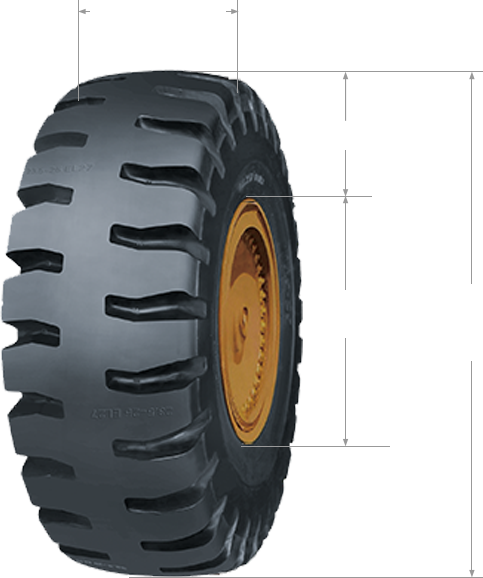1. 225
2. /
3. 40
4. Z
5. R
6. 18
7. 92
8. W
• Speed Symbol
• Nominal Rim Diameter in Inches
• Initial Z means speed capacity ≥ 240Kph
• Nominal Aspect Ratio as a Percentage
• Nominal Section Width in Millimeters
Aspect ratio(%) = Seciton Height Seciton Width X 100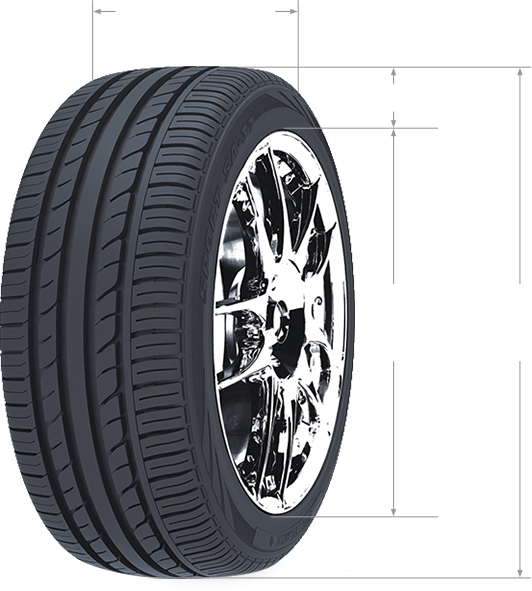1. overall width
2. section height (H)
3. rim
diameter
4. overall
diameter
1. 385
2. /
3. 65
4. R
5. 22.5
6. 158
7. L
• Speed Symbo
• Nominal Rim Diameter in Inches
• Nominal Aspect Ratio as a Percentage
• Nominal Section Width in Millimeters
Aspect ratio(%) = Seciton Height Seciton Width X 100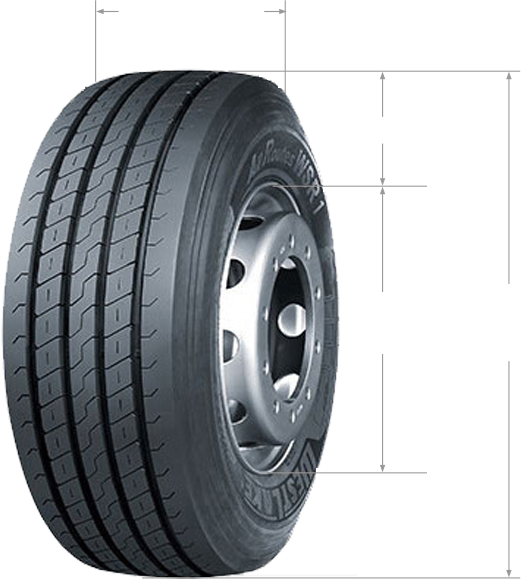1. overall width
2. section height (H)
3. rim
diameter
4. overall
diameter
1. 27.00
2. R
3. 49
4.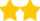• Star Rating
• Rim Diameter (inches)
• Section Width (inches)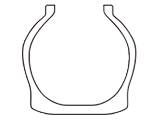SH SW = 0.95
###### Regulars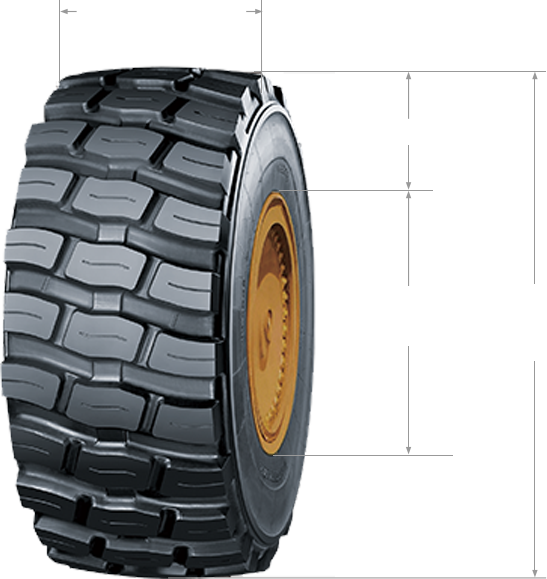1. overall width
2. section height (H)
3. rim
diameter
4. overall
diameter
1. 29.5
2. R
3. 25
4.• Star Rating
• Rim Diameter (inches)
• Section Width (inches)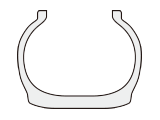SH SW = 0.80
###### Wide Base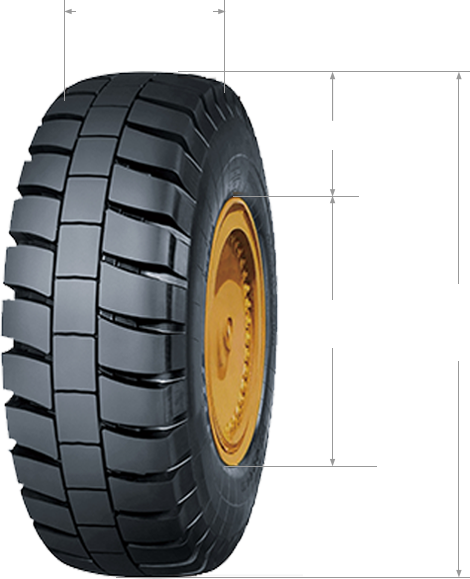1. overall width
2. section height (H)
3. rim
diameter
4. overall
diameter
1. 35
2. /
3. 65R
4. 33
5.• Star Rating
• Rim Diameter (inches)
• Aspect Ratio 65 Series
• Section Width (inches)SH SW = 0.65
1. 170
2. E
3. 385
4. /
5. 95
6. R
7. 24
• Rim Diameter (inches)
• Aspect Ratio 95 Series
• Section Width (mm)
• Speed Symbol